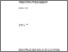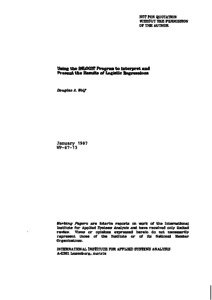Using the INLOGIT Program to Interpret and Present the Results of Logistic Regressions

Wolf, D.A. (1987). Using the INLOGIT Program to Interpret and Present the Results of Logistic Regressions. IIASA Working Paper. IIASA, Laxenburg, Austria: WP-87-013Preview
Text
WP-87-013.pdf

Abstract

Nonlinear statistical models, of which logistic regression is one example, are often used in applied demographic analysis as well as in many other social sciences. Presentation of the results of such models can be enhanced by the calculation of predicted probabilities, or expected values of the phenomenon of interest, yet such calculations can often be rather time-consuming. This paper describes the use of a computer program, INLOGIT, which can help the applied researcher interpret and display the results of a logistic regression model.

Item Type: Monograph (IIASA Working Paper) World Population (POP) IIASA Import 15 Jan 2016 01:58 27 Aug 2021 17:13 http://pure.iiasa.ac.at/3038View Item